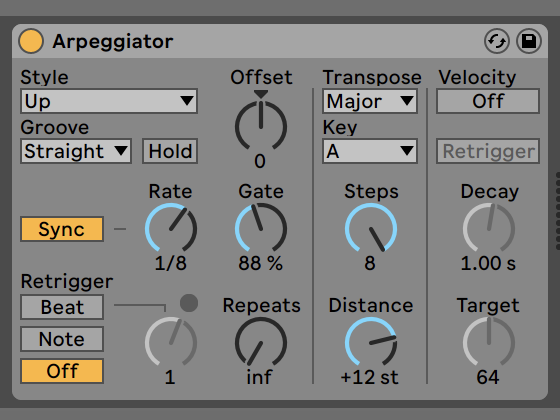# Key intervals value

hey everyone, I have been trying to reproduce some of Ableton Arpegiator functions and got stuck with the key transpose section, not sure about what value to multiply the key by to find the same key as in the screenshot posted.when observed on the spectro freq I see that the root which is 64.midicps (E4) goes to C#6 which is 85.midicps

when divided 85.midicps/64.midicps I get 3.3635856610149

after the key goes to A7 (105.midicps) but when I divide

105.midicps/85.midicps I get 3.1748021039364

and after it goes to F9# (126.midicps)

and once divided 126.midicps/105.midicps I get back the 3.3635856610149

is there a built in function to get theses intervals correct?

thanks a lot

Not sure if I understand your question correctly, but midi note numbers (e.g. 60) are linearly spaced. So you just need to add stuff to it, not multiply it. Frequencies on the other hand (e.g. 60.midicps) are exponentially spaced and require multiplications.

If you work in midinumbers, you don’t have to multiply, you simply have to add.
Adding 1 to a midinote number transposes the note up with a semitone.

So to transpose something in the key of C major (60 is a “c” note) to the key of A major (69 is an “a” note) you simply add 9 (= 69-60) to all midi note numbers.

You are comparing different intervals, so that’s why the frequency ratios are different (105 - 85 = 20. 85 - 64 = 21).

You may benefit from the .midiratio method as well…

``````21.midiratio
``````

gives the same result as the calculation in your code

thanks a lot Shiihs and PitchTrebler!

I got quite confused about it myself and ended hard coding each key of the output to SC, I indeed tried using .midicps and I will look further into .midiratio and other suggestion.

thanks